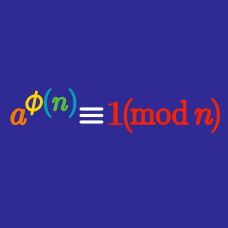Number Theory

# Fermat's Little Theorem

Fermat's little theorem states that if $a$ and $p$ are coprime positive integers, with $p$ prime, then $a^{p-1} \bmod p = 1$.

Which of the following congruences satisfies the conditions of this theorem?

\begin{aligned} 1^4 \bmod 5 &=& 1 \\ 2^4 \bmod 5 &=& 1 \\ 3^4 \bmod 5 &=& 1 \\ 4^4 \bmod 5 &=& 1 \end{aligned}

We are given that the 4 congruences above are true. Is the following congruence true as well?

$5^4 \bmod 5 = 1$

True or false?

$42^6 \bmod 7 = 1.$

What is the remainder when $3^{456}$ is divided by 7?

$\large 32^{23} \pmod {23} =\, ?$

×